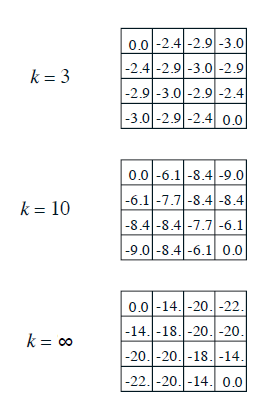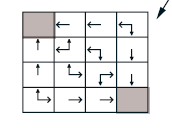Chapter 1 : Introduction
Chapter 2 : Markov Decision Process
Chapter 3 : Bellman Equation
Chapter 4 : Dynamic Programming
CHAPTER 5: Monte-Carlo Methods
CHAPTER 6 : Temporal Difference Methods
CHAPTER 7 : Off-Policy Control
CHAPER 8: Value Function Approximatiobn
CHAPTER 9 : DQN(Deep Q-Networks)
Policy Iteration
앞으로 순서대로 Dynamic programming, Monte-carlo methods, Temporal difference methods를 살펴볼 것입니다. 세 방법에 대해서 Sutton교수님은 책에서 다음과 같이 설명하고 있습니다.
dynamic programming, Monte Carlo methods, and temporal-difference learning. Each class of methods has its strengths and weaknesses. Dynamic programming methods are well developed mathematically, but require a complete and accurate model of the environment. Monte Carlo methods don't require a model and are conceptually simple, but are not suited for step-by-step incremental computation. Finally, temporal-difference methods require no model and are fully incremental, but are more complex to analyze. The methods also differ in several ways with respect to their efficiency and speed of convergence.
또한 Dynamic Programming을 다음과 같이 정의하고 있습니다.
The term dynamic programming (DP) refers to a collection of algorithms that can be used to compute optimal policies given a perfect model of the environment as a Markov decision process (MDP)

### Planning vs Learning

본격적으로 Dynamic Programming에 대해서 설명하기 전에 Planning과 Learning의 차이를 먼저 보도록 하겠습니다. 간단하게 말하자면 Planning이란 environment의 model을 알고서 문제를 푸는 것이고, Learning이란 environment의 model을 모르지만 상호작용을 통해서 문제를 푸는 것을 말합니다. Dynamic Programming은 Planning으로서 Environment의 model(reward, state transition matrix)에 대해서 안다는 전제로 문제를 푸는 방법(Bellman equation을 사용해서)을 말합니다. 강화학습과는 planning과 learning으로서 그 분류가 다르지만 강화학습이 이 DP(Dynamic programming)에 기반을 두고서 발전했기 때문에 DP를 이해하는 것이 상당히 중요합니다.### Prediction & Control

Dynamic Programming은 다음 두 step으로 나뉩니다. (1) Prediction (2)Control입니다. 이름은 중요하지 않고 이를 통해서 DP가 어떻게 작동하는지 대충 감을 잡으면 좋을 것 같습니다. 즉 현재 optimal하지 않는 어떤 policy에 대해서 value function을 구하고(prediction) 현재의 value function을 토대로 더 나은 policy를 구하고 이와 같은 과정을 반복하여 optimal policy를 구하는 것입니다.### Policy evaluationPolicy evaluation은 prediction 문제를 푸는 것으로서 현재 주어진 policy에 대한 true value functiond을 구하는 것이고 Bellman equation을 사용합니다. 현재 Policy를 가지고 true value function을 구하는 것은 one step backup으로 구합니다.
이전의 Bellman equation의 그림과 다른 점은 value function에 k라는 iteration숫자가 붙은 것입니다.현재 상태의 value function을 update하는데 reward와 next state들의 value function을 사용하는 것 입니다. 전체 MDP의 모든 state에 대해서 동시에 한 번씩 Bellman equation을 계산해서 update함으로서 k가 하나씩 올라가게 됩니다. 차례 차례 state 별로 구하는 것이 아니고 한 번에 계산해서 한 번에 value function을 update합니다. 이 Policy evaluation과정을 예를 통해서 설명해보겠습니다.
4X4 gridworld example입니다. state는 회색으로 표시된 terminal state가 두 개 있고 nonterminal state가 14개 있습니다. action은 상,하,좌,우 네 개를 취할 수 있고 time step이 지날 때 마다 -1의 reward를 받습니다. 따라서 agent는 reward를 최대로 하는 것이 목표이기 때문에 terminal state로 가능한 한 빨리 가려고 할 것입니다. 그러한 policy를 계산해내는 것이 DP이고 DP는 evaluation과 improve 두 단계로 나눠지게 됩니다. evaluation은 말 그대로 현재의 policy가 얼마나 좋은가를 판단하는 것이고 판단 기준은 그 policy를 따라가게 될 경우 받게 될 value function입니다. 처음 policy는 uniform random policy로서 모든 state에서 똑같은 확률로 상하좌우로 움직이는 policy입니다. 이 policy를 따라갈 경우 얻게 될 value function을 계산해보도록 하겠습니다.처음 시작은 아래와 같은 random policy로 시작합니다.DP에서 value function은 Bellman equation을 통해서 구한다고 말했었습니다. 밑의 그림에서 k는 iteration number를 의미하고 one step씩 각 state의 value function을 update하는 과정입니다.한 스텝씩 다음 식을 통해서 value function을 update합니다.k=1을 보면 nonterminal state의 V = 4 X 0.25(-1 + 0) = -1이 됩니다. k=2에서 (1,2)state를 보면 v = 1 X 0.25(-1 + 0) + 3 X 0.25(-1 + -1) = -1.7(소수 1째 자리까지만 표현) (1,2)state에서 위로 action을 취하면 벽에 부딪히는데 그러면 자신의 state로 돌아오게 됩니다. 따라서 up, right, down의 action에 의해서 agent는 각각 -1의 value function을 가진 state에 도달합니다.
이런 식으로 무한대까지 계산하게 되면 현재 random policy에 대한 true value function을 구할 수 있고 이러한 과정을 policy evaluation이라고 합니다.

### Policy iteration

해당 policy에 대한 참 값을 얻었으면 이제 policy를 더 나은 policy로 update해줘야 합니다. 그래야 점점 optimal policy에 가까워질 것입니다. 그러한 과정을 Policy improvement라고 합니다. improve하는 방법으로는 greedy improvement가 있습니다. 다음 식에서 보듯이 간단히 다음 state중에서 가장 높은 value function을 가진 state로 가는 것입니다. 즉, max를 취하는 것입니다.위와 같이 evaluation을 통해 구한 value function을 토대로 한 번 improve를 하게 되면 다음과 같이 됩니다.상당히 작은 gridworld이기 때문에 evaluation 한 번 improve 한 번 하면 optimal policy(위 그림)을 구할 수가 있는데 보통은 이러한 과정을 계속 반복해줘야 optimal policy를 구할 수 있습니다. 이러한 반복되는 과정을 Policy Itertion이라고 합니다. 다음과 같이 그림으로 나타낼 수 있습니다.하지만 방금 했던 예제에서도 알 수 있듯이 꼭 무한대로 evaluation을 해줘야하는 것은 아닙니다. evaulation의 iteration을 세 번만 해도 결국에는 optimal policy를 구할 수 있으며 단 한 번만 해줘도 optimal policy를 구할 수 있고 그러한 방법을 value iteration이라고 합니다.